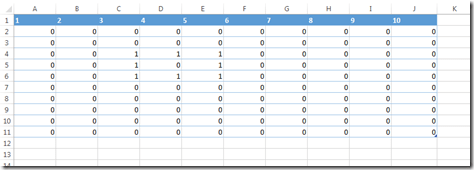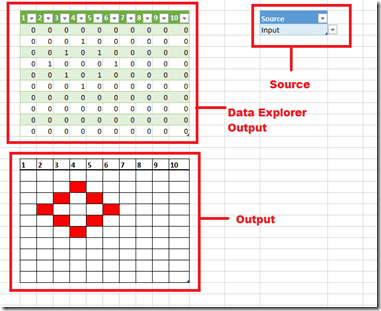# A Partly Successful Attempt To Create Life With Data Explorer (Power Query)

NOTE: This post was written before Data Explorer was renamed as Power Query. All of the content is still relevant to Power Query.

I’ll apologise for the title right away: this post isn’t about a Frankenstein-like attempt at creating a living being in Excel, I’m afraid. Instead, it’s about my attempt to implement Jon Conway’s famous game ‘Life’ using Data Explorer, how it didn’t fully succeed and some of the interesting things I learned along the way…

When I’m learning a new technology I like to set myself mini-projects that are more fun than practically useful, and for some reason a few weeks ago I remembered ‘Life’ (which I’m sure almost anyone who has learned programming has had to write a version of at some stage), so I began to wonder if I could write a version of it in Data Explorer. This wasn’t because I thought Data Explorer was an appropriate tool to do this – there are certainly better ways to implement Life in Excel – but I thought doing this would help me in my attempts to learn Data Explorer’s formula language and might also result in an interesting blog post.

Here’s the code I came up with eventually:

`let`

`    Source = Excel.CurrentWorkbook(){[Name="Input"]}[Content],`

`    GetAction = Excel.CurrentWorkbook(){[Name="Source"]}[Content]{0}[Source],`

`    GetLastOutput = try Excel.CurrentWorkbook(){[Name="Output"]}[Content],`

`    ChooseInput = if GetAction="Input" or GetLastOutput[HasError]`

`        then Source else GetLastOutput[Value],`

`    Unpivot = Table.Unpivot(ChooseInput,{"1", "2", "3", "4", "5", "6"`

`        , "7", "8", "9", "10"},"Column","Value"),`

`    InsertedIndex = Table.AddIndexColumn(Unpivot,"Index"),`

`    PivotedRows = Table.RowCount(InsertedIndex),`

`    OriginalColumnCount = Table.ColumnCount(ChooseInput),`

`    GetValue = (i) =>; if i<0 or i>=PivotedRows then 0 `

`        else InsertedIndex[Value]{i},`

`    GetNeighbours = (i) =>; GetValue(i-1) + GetValue(i+1) `

`        + GetValue(i-OriginalColumnCount) + GetValue(i+OriginalColumnCount)`

`        + GetValue(i-OriginalColumnCount-1) + GetValue(i-OriginalColumnCount+1)`

`        + GetValue(i+OriginalColumnCount-1) + GetValue(i+OriginalColumnCount+1),`

`    NeighbourCount = Table.AddColumn(InsertedIndex, "Neighbours",`

`        each GetNeighbours([Index])),`

`    Custom1 = Table.AddColumn(NeighbourCount , "NewValue",`

`        each if [Value]=1 and ([Neighbours]=2 or [Neighbours]=3)`

`        then 1 else if [Value]=0 and [Neighbours]=3 then 1 else 0),`

`    HiddenColumns = Table.RemoveColumns(Custom1,{"Value", "Index", "Neighbours"}),`

`    Custom2 = Table.Group(HiddenColumns, {"Column"},`

`        {{"Count", each Table.Transpose(Table.RemoveColumns(_,"Column")), type table}}),`

`    #"Expand Count" = Table.ExpandTableColumn(Custom2, "Count", {"Column1", "Column2"`

`        , "Column3", "Column4", "Column5", "Column6", "Column7", "Column8", "Column9"`

`        , "Column10"}, {"1", "2", "3", "4", "5", "6", "7", "8", "9","10"}),`

`    HiddenColumns1 = Table.RemoveColumns(#"Expand Count",{"Column"})`

`in`

`    HiddenColumns1`

Some explanation of the code:

• The first problem that I faced was that in Life you need to start with a grid of cells, apply the rules of the game, and then output the results to the same grid. This is a problem for Data Explorer because, unless you have already run a query, the output table doesn’t exist, and even if you have you can’t update the values in that table without them being overwritten. So I decided to create a table where you can enter the initial state of your grid as a series of 1s and 0s. It’s on the Input sheet and its called Input:• Whether the Input table is used as the starting point for the query depends on the contents of yet another table, called Source, on the sheet called Output in the workbook. It can hold one of two text values: “Input” or “Output” (I used Excel’s Data Validation functionality to lock this down), and the ChooseInput step then determines whether the Input table or the table called Output is used as the input for the Data Explorer query. Here’s what the Output worksheet looks like:The workflow is therefore as follows: enter the starting point on the Input table, make sure the Source table shows “Input”, refresh the query, change the Source table to “Output” and then refresh the query to show each subsequent iteration.

• The Output table simply displays the same values as the table that shows the results of the Data Explorer query. I created a separate table for two reasons: one, I wanted to use custom formatting to show the results; and two, to try to work around the big problem that I eventually found I couldn’t work around completely, which is that Data Explorer doesn’t actually support this type of recursive query (ie scenarios where the output of a query is also used as the input). This thread on the Data Explorer MSDN Forum has some details on the problem. I found I got errors after just about every other step when using the Data Explorer-created table as the input, whereas the errors were much less frequent if I duplicated the values in a separate table. It now works most of the time, but it still errors far too much for my liking. Hohum.
• I did also use Data Explorer’s error-handling functionality, using the try statement in the GetLastOutput step, to solve this problem but it made no difference. It was good to find out how a try statement works: basically when you use it, the return value is a record containing two values, one which indicates whether an error occurred in the try, and the other the value that was returned if there was no error. You can see me checking these values in the ChooseInput step, with the calls to GetLastOutput[HasError] (which returns true if there was an error in the try) and GetLastValue[Value] (which returns the value tested in the try if there was no error).
• Once the query worked out which input to use, the next step is to calculate the values in the grid for the next iteration. I decided that the easiest way to do this was to unpivot the resultset using the the new UnPivot() function; with all the data in a single column it was then relatively easy to declare the functions GetValue() and GetNeighbours() to apply the rules of Life, output the results in a new column in the Custom1 step.
• The final problem to solve was that I needed to re-pivot the data to get it back into original table format. Although Data Explorer has an UnPivot() function it doesn’t have a Pivot() function; luckily, Peter Qian showed me how to do this on the forum in this thread using Table.Group() and my code is in the Custom2 step.

So despite the errors (and Data Explorer is still in beta, so some errors are only to be expected), many lessons learned. I hope you find this useful too.

### 5 responses

1.Marco Russo says:

Very nice demo. It shows many capabilities of Data Explorer that I wasn’t aware of!

This site uses Akismet to reduce spam. Learn how your comment data is processed.